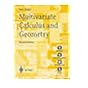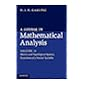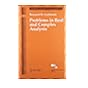Normal view MARC view ISBD view

# A course in mathematical analysis / Vol. II D. J. H. Garling. metric and topological spaces, functions of a vector variable.

Material type:TextPublisher: India : Cambridge University Press, 2015Edition: first south asian edition.Description: x, 617 pages, vol. 2 ; 26 cm.Content type: text Media type: unmediated Carrier type: volumeISBN: 9781107032033 (hardback); 9781107519039 (paperback).DDC classification: 514/.325 Other classification: MAT034000 Online resources: Cover image
Incomplete contents:
v. 2. Metric and topological spaces, functions of a vector variable
Machine generated contents note: Introduction; Part I. Metric and Topological Spaces: 1. Metric spaces and normed spaces; 2. Convergence, continuity and topology; 3. Topological spaces; 4. Completeness; 5. Compactness; 6. Connectedness; Part II. Functions of a Vector Variable: 7. Differentiating functions of a vector variable; 8. Integrating functions of several variables; 9. Differential manifolds in Euclidean space; Appendix A. Linear algebra; Appendix B. Quaternions; Appendix C. Tychonoff's theorem; Index.
Summary: "The three volumes of A Course in Mathematical Analysis provide a full and detailed account of all those elements of real and complex analysis that an undergraduate mathematics student can expect to encounter in their first two or three years of study. Containing hundreds of exercises, examples and applications, these books will become an invaluable resource for both students and teachers. Volume I focuses on the analysis of real-valued functions of a real variable. This second volume goes on to consider metric and topological spaces. Topics such as completeness, compactness and connectedness are developed, with emphasis on their applications to analysis. This leads to the theory of functions of several variables: differentiation is developed in a co-ordinate free way, while integration (the Riemann integral) is established for functions defined on subsets of Euclidean space. Differential manifolds in Euclidean space are introduced in a final chapter, which includes an account of Lagrange multipliers and a detailed proof of the divergence theorem. Volume III covers complex analysis and the theory of measure and integration. "-- Provided by publisher.
List(s) this item appears in: 2015-05-05
Item type Current location Call number Status Date due Barcode Item holds
Book Chennai Mathematical Institute
General Stacks
515 GAR (Browse shelf) Checked out 11/10/2022 9516
Total holds: 0
##### Browsing Chennai Mathematical Institute Shelves , Shelving location: General Stacks Close shelf browserNo cover image available No cover image availableNo cover image available No cover image available515 DIN Multivariate calculus and geometry : Seán Dineen. 515 FEF Advances in analysis : the legacy of Elias M. Stein / 515 GAR A course in mathematical analysis / Vol. I foundations and elementary real analysis. 515 GAR A course in mathematical analysis / Vol. II metric and topological spaces, functions of a vector variable. 515 GAR A course in mathematical analysis / Vol. III complex analysis, measure and integration. 515 GEL Problems in real and complex analysis / 515 GHO A course in calculus and real analysis /